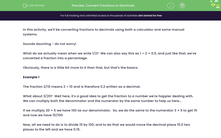# Convert Fractions to Decimals

In this worksheet, students will convert fractions to decimals.Key stage:  KS 3

Curriculum topic:   Number

Curriculum subtopic:   Work Interchangeably with Terminating Decimals and Fractions

Popular topics:   Fractions worksheets, Decimals worksheets

Difficulty level:#### Worksheet Overview

In this activity, we'll be converting fractions to decimals using a variety of methods.

Sounds daunting - do not worry!What do we actually mean when we write 1/2?

It means 1 ÷ 2 = 0.5, and just like that, we've converted a fraction into a decimal!

Obviously, there is a little bit more to it than that, but that's the basics.

Example 1

The fraction 2/10 means 2 ÷ 10

Can you remember how to divide a decimal by 10? We move the digits one place to the right, and the number 2 becomes 0.2

What about 3/20?  Well here, it's a good idea to get the fraction to a number we're happier dealing with.  We can multiply both the denominator and the numerator by the same number to help us here...

If we multiply 20 × 5 we have 100 as our denominator.  So, we do the same to the numerator 3 × 5 to get 15 and now we have 15/100

Now, all we need to do is to divide 15 by 100, and to do that we would move the digits two places to the right and we have 0.15.

But, what do we do if we have a number that can't easily be changed so that the numerator is 100?  What about 2/7?

Example 2

This fraction seems a bit more of a challenge, so we can tackle it in one of two ways. It can be calculated immediately with a calculator, but can also be found manually by dividing.This shows that 2/7 as a decimal is 0.285714.....

This rounds to 0.2857 to 4 decimal places.

So, you can see that there are many ways of doing these division sums. Often, you could just use a calculator but it is important to know how to do the other methods for times when a calculator is either not allowed, or not available.

Are you ready to get started?

### What is EdPlace?

We're your National Curriculum aligned online education content provider helping each child succeed in English, maths and science from year 1 to GCSE. With an EdPlace account you’ll be able to track and measure progress, helping each child achieve their best. We build confidence and attainment by personalising each child’s learning at a level that suits them.

Get started